## Selina Concise Mathematics Class 10 ICSE Solutions Chapter 2 Banking (Recurring Deposit Accounts) Ex 2B

These Solutions are part of Selina Concise Mathematics Class 10 ICSE Solutions. Here we have given Selina Concise Mathematics Class 10 ICSE Solutions Chapter 2 Banking Ex 2B.

Other Exercises

Question 1.
Pramod deposits ₹ 600 per month in a Recurring Deposit Account for 4 years. If the rate of interest is 8% per year; calculate the maturity value of his account.
Solution:
Deposit per month (P) = ₹ 600
Rate of interest (r) = 8%
Period (n) = 4 years = 48 months.
According to formula,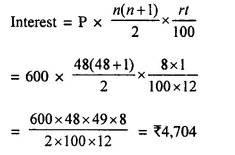Maturity value = ₹ 600 x 48 + ₹ 4,704 = ₹ 28,800 + ₹ 4,704 = ₹ 33504

Question 2.
Ritu has a Recurring Deposit Account in a bank and deposits ₹ 80 per month for 18 months. Find the rate of interest paid by the bank if the maturity value of this account is ₹ 1,554.
Solution:
Let rate of interest = r%,
n = 18,
P = ₹ 80
and A is maturity value.
Using formulaQuestion 3.
The maturity value of a R.D. Account is ₹ 16,176. If the monthly installment is ₹ 400 and the rate of interest is 8%; find the time (period) of this R.D. Account.
Solution:
Here maturity value (A) = ₹ 16,176
Rate = 8%,
P = ₹ 400
Let period = n (No. of months)
Using formula :
I = A – P x n = 16,176 – 400 x n = 16,716 – 400n.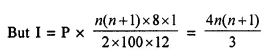⇒ 48,528 – 1,200n = 4n² + 4n
⇒ 4n² + 4n + 1200n – 48,528 = 0
⇒ 4n² + 1,204n – 48,528 = 0
⇒ n² + 301n — 12,132 = 0 (dividing by 4)
⇒ n² – 36n + 337n – 12,132 = 0
⇒ n (n – 36) + 337 (n – 36) = 0
⇒ (n – 36) (n + 337) = 0
Either n = 36 months or n = -337, which is not possible.
Time = 36 months = 3 years

Question 4.
Mr. Bajaj needs ₹ 30,000 after 2 years. What least money (in multiple of ₹ 5) must he deposit every month in a recurring deposit account to get required money at the end of 2 years, the rate of interest being 8% p.a. ?
Solution:
Amount of maturity = ₹ 30000
Period (n) = 2 years = 24 months
Rate = 8% p.a.
Let x be the monthly depositAmount of monthly deposit in the multiple of ₹ 5 = ₹ 1155

Question 5.
Rishabh has a recurring deposit account in a post office for 3 years at 8% p.a. simple interest. If he gets ₹ 9,990 as interest at the time of maturity, find :
(i) the monthly installment.
(ii) the amount of maturity.
Solution:
Total interest = ₹ 9990
Period (n) = 3 years = 36 months
Rate of interest (r) = 8%
(i) Let monthly installment = xMonthly installment = ₹ 2250
(ii) Amount of maturity = Principal + Interest
= 36 x 2250 + 9990
= ₹ 81000 + 9990 = ₹ 90990

Question 6.
Gopal has a cumulative deposit account and deposits ₹ 900 per month for a period of 4 years. If he gets ₹ 52,020 at the time of maturity, find the rate of interest.
Solution:
Maturity value = ₹ 52,020
Monthly installment (P) = ₹ 900
Total principal = ₹ 900 x 48 = ₹ 43200
Amount of interest = ₹ 52020 – ₹ 43200 = ₹ 8820
Let rate of interest = r%Question 7.
Deepa has a 4 year recurring deposit account in a bank and deposits ₹ 1,800 per month. If she gets ₹ 1,800 per month. If she gets ₹ 1,08,450 at the time of maturity, find the rate of interest.
Solution:
Deposit per month = ₹ 1800
Period = 4 years = 48 months
Maturity value = ₹ 108450
Total principal = ₹ 1800 x 48 = ₹ 86400
Amount of interest = ₹ 108450 – 86400 = ₹ 22050
Let r be the rate of interest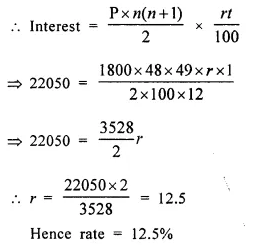Question 8.
Mr. Britto deposits a certain sum of money each month in a Recurring Deposit Account of a bank. If the rate of interest is of 8% per annum and Mr. Britto gets ₹ 8,088 from the bank after 3 years, find the value of his monthly installment. (2013)
Solution:
Let monthly installment = ₹ x
Period (n) = 3 x 12 months = 36 months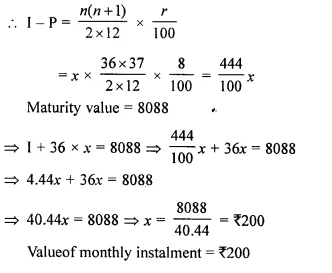Question 9.
Sharukh opened a Recurring Deposit Account in a bank and deposited ₹ 800 per month for 1$$\frac { 1 }{ 2 }$$ years. If he received ₹ 15,084 at the time of maturity, find the rate of interest per annum. (2014)
Solution:
Money deposited per month (P) = ₹ 800
r = ?Question 10.
Katrina opened a recurring deposit account with a Nationalised Bank for a period of 2 years. If the bank pays interest at the rate of 6% per annum and the monthly installment is ₹ 1,000, find the :
(i) interest earned in 2 years
(ii) maturity value. (2015)
Solution:
Period (n) = 2 years = 2 x 12 = 24 months
Rate of interest (r) = 6%
Monthly installment (P) = ₹ 1000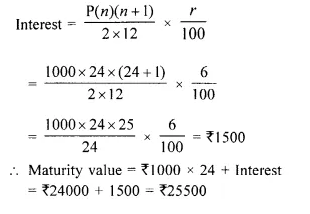Question 11.
Mohan has a recurring deposit account in a bank for 2 years at 6% p.a. simple interest. If he gets ₹ 1200 as interest at the time of maturity, find :
(i) the monthly installment
(ii) the amount of maturity
Solution:
(i) Interest = ₹ 1200,
n = 2 x 12 = 24 months,
r = 6%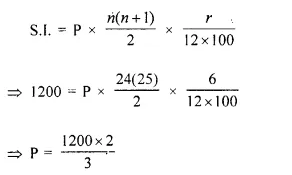⇒ P = ₹ 800
So the monthly installment is ₹ 800
(ii) Total sum deposited = P x n = ₹ 800 x 24 = ₹ 19200
The amount that Mohan will get at the time of maturity = Total sum deposited + Interest Received
= ₹ 19200 + ₹ 1200 = ₹ 20400
Hence, the amount of maturity is ₹ 20400

Hope given Selina Concise Mathematics Class 10 ICSE Solutions Chapter 2 Banking Ex 2B are helpful to complete your math homework.

If you have any doubts, please comment below. Learn Insta try to provide online math tutoring for you.